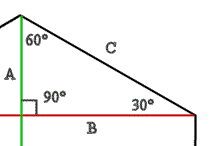# Drawing a Hexagon in Processing / Java

As part of my little HarmonicTable project I spent a lot of time figuring out how to draw a proper hexagon. It seems I wasn’t alone in this endeavor, as I’ve received a few comments and e-mails about how exactly to do this. At the request of others I’ve moved the Hexagon class back into Processing, and written a dead simple PDE sketch to show you how to use it.

First, lets look at the constructor for the Hexagon class:

``````public Hexagon(Object p,
int newStartX,
int newStartY,
int sideLength){
if (p instanceof PGraphics)
buffer = (PGraphics) p;
if (p instanceof PApplet)
parent = (PApplet) p;

setStartX(newStartX);
setStartY(newStartY);
c = sideLength;
a = c/2;
}
``````

The constructor takes 4 parameters:

• Object p: This is where you want the object to be drawn, you should just pass in ‘this’ in PDE.
• newStartX, newStartY: The starting coordinates of the hexagon. You may pass in 0 for both if you are going to translate the screen yourself.
• sideLength: This tells us how big to draw the hexagon based on the length of one side

Based on these values we make some calculations for the rest of the sides. Here is a picture illustrating the sides we’re calculating so you can get a better idea of what they actually mean.Now that we have an object with all of the side lengths calculated, its simply a matter of drawing this to the screen (or buffer), using the drawHex function:

``````//draw hex shape
parent.beginShape();
parent.vertex(0,b);
parent.vertex(a,0);
parent.vertex(a+c,0);
parent.vertex(2*c,b);
parent.vertex(a+c,2*b);
parent.vertex(a,2*b);
parent.vertex(0,b);
parent.endShape();
}
``````

This code just looks at the a,b, and c coordinates we’ve stored in the object, and creates a shape with the vertices at the correct points.

If you import the Hexagon class into processing, using it is easy. Here is what a simple sketch to draw a hexagon would look like:

``````Hexagon hexagon;

void setup(){
hexagon = new Hexagon(this, 10, 10, 15);
}

void draw(){
hexagon.drawTranslatedHex();
}
``````

Easy! From there you can create an array of Hexagons if you like, with x and y coordinates that draw them wherever you like. If you want to make the translations yourself, and apply fill and stroke values, you’ll want to use the drawHex() function directly, and move about the screen wherever you like. If you’re unfamiliar with translations in processing you should check out this reference.